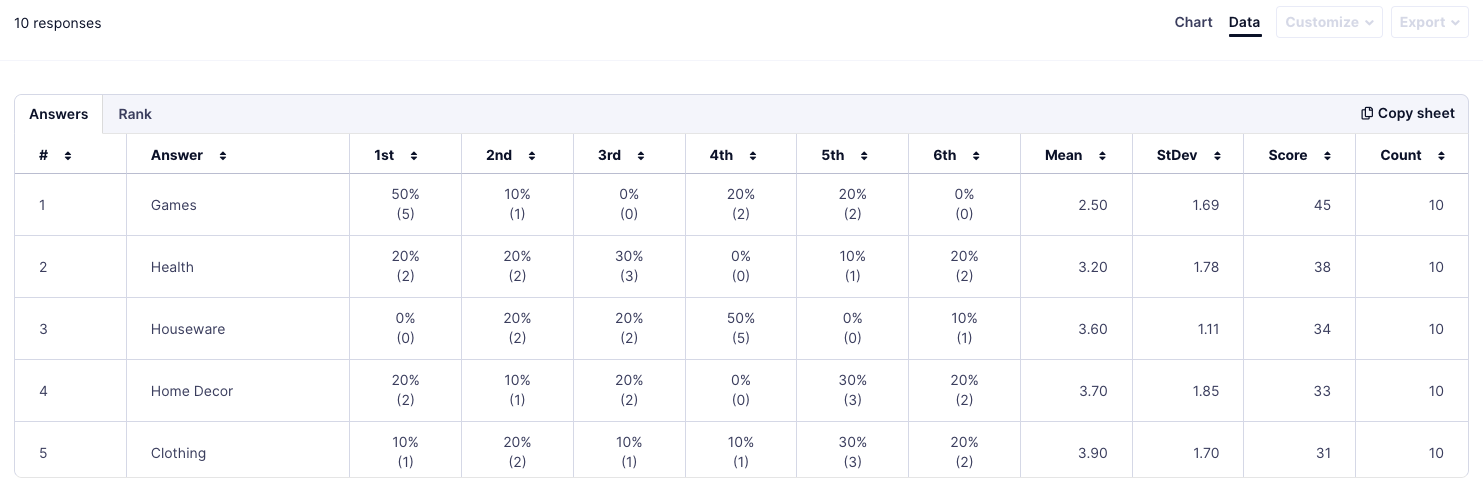# Understand Rank Order (Drag & Drop) question results

Learn how to find and understand Rank Order (Drag & Drop) question results.

Plan Availability: Essentials, Professional, International, and Premium
👥 User roles: Owner, Admins, and Researchers

## About Rank Order (Drag & Drop) question results

• Find data collected by Rank Order (Drag & Drop) questions in the Results section of your study.
• There are various charts and tables available to view results.
• The chart below shows the total score obtained by each item.
• This score is a sum of the points it gets from each participant. For example, in a rank order question with 6 ranking points:
• Items ranked First = 6 points
• Items ranked Second = 5 points
• Items ranked Third = 4 points
• Items ranked Fourth = 3 points
• Items ranked Fifth = 2 points
• Items ranked Sixth = 1 point• The Answers table shows the ranking order percentage of participant answers for each item in each position.
• The number in parenthesis is the number of times that participants placed that item in the position.
• 1st, 2nd, 3rd, 4th, 5th, and 6th: The ranking positions for each answer.
• Mean: The average position of the item in the total ranking.
• Stdev:  Standard deviation is the difference between the value of an observation and the mean.
• Score: The total score of each item.
• Count: The total number of participants who responded.## Rank table

The Rank table shows items included in each ranking position in descending order.